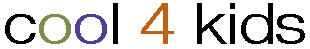Looking for something in particular? the entire directory   only this category More search optionsHome: School Time: Math
CATEGORIES:Algebra (3)
 Arithmetic (18)
 Calculus (2)
 Counting and Numbers (2)
 Geometry (3)
 Mathematicians (16)
 Roman Numerals (0)
 Statistics (0)
 Trigonometry (3)
LINKS:Pages: 1 2 [>>]
• Coolmath4kids  pop
Math games, problems, calculators, puzzles, and fun and for all levels.
(Added: Mon Jan 01 2001 Hits: 1523 Rating: 0.00 Votes: 0)   Rate It   Review It
• A+ Math
Helps students improve their math skills interactively.
(Added: Mon Jan 01 2001 Hits: 765 Rating: 0.00 Votes: 0)   Rate It   Review It
• AAA Math
Hundreds of online interactive arithmetic lessons, problems, and games for grades K-8.
(Added: Mon Jan 01 2001 Hits: 746 Rating: 0.00 Votes: 0)   Rate It   Review It
• Activities in Math
Interactive activities and quizzes on topics such as basic arithmetic, algebra, and trigonometry. Also includes brain teasers and puzzles.
(Added: Mon Jan 01 2001 Hits: 689 Rating: 0.00 Votes: 0)   Rate It   Review It
• Allmath.com
Features free flashcards, online games, biographies of mathmaticians, and an Ask-the-Experts service.
(Added: Mon Jan 01 2001 Hits: 693 Rating: 0.00 Votes: 0)   Rate It   Review It
A question and answer service for K-12 math students. Includes a searchable archive, FAQ section, plus a history of math through a look at some of the most famous problems mathematicians have ever tackled.
(Added: Mon Jan 01 2001 Hits: 737 Rating: 0.00 Votes: 0)   Rate It   Review It
• Brain Teasers
Offers new math puzzles each week. Designed for students in grades 3-7.
(Added: Mon Jan 01 2001 Hits: 671 Rating: 0.00 Votes: 0)   Rate It   Review It
• Brain Teasers and Math Puzzles
Interactive puzzles to challenge logic and mathematical skills. Immediate feedback with detailed solutions is provided.
(Added: Mon Jan 01 2001 Hits: 617 Rating: 0.00 Votes: 0)   Rate It   Review It
• Convertalot
A collection of free Java-based measurements converters, calculators, unit translators, and games.
(Added: Mon Jan 01 2001 Hits: 636 Rating: 0.00 Votes: 0)   Rate It   Review It
• Dositey.com
Interactive lessons and exercises, open-ended questions, printable worksheets, and challenging problems sorted by topic and grade range.
(Added: Mon Jan 01 2001 Hits: 700 Rating: 0.00 Votes: 0)   Rate It   Review It
Helps students work through the six most common stumbling blocks to working with fractions. Also explains how to find the least common denominator, how to identify equivalent fractions, and how to reduce and rename fractions.
(Added: Mon Jan 01 2001 Hits: 763 Rating: 0.00 Votes: 0)   Rate It   Review It
• Free Math Help Online
My Math Man gives free algebra and geometry 2-3 minutes audio video lessons from textbooks you use in class with interactive practice.
(Added: Mon Jan 01 2001 Hits: 608 Rating: 0.00 Votes: 0)   Rate It   Review It
• Homework Help at Math Goodies
Math lessons with a problem-solving approach. Includes a homework help message board for help with specific problems.
(Added: Mon Jan 01 2001 Hits: 676 Rating: 0.00 Votes: 0)   Rate It   Review It
• K-8 Math Lessons
Contains a large series of math lessons for grades K-8. Each lesson opens with a short explanation of the concept, provides a series of interactive exercises, then ends with a related review game.
(Added: Mon Jan 01 2001 Hits: 577 Rating: 0.00 Votes: 0)   Rate It   Review It
• Math Goodies
Features interactive math lessons, homework help, worksheets, puzzles, message boards, and newsletters.
(Added: Mon Jan 01 2001 Hits: 733 Rating: 0.00 Votes: 0)   Rate It   Review It
• Math in Daily Life
Virtual exhibit looks at the ways people use math every day, from cooking to planning for retirement.
(Added: Mon Jan 01 2001 Hits: 549 Rating: 0.00 Votes: 0)   Rate It   Review It
• Math Worksheets
Features printable worksheets for students to practice with.
(Added: Mon Jan 01 2001 Hits: 551 Rating: 0.00 Votes: 0)   Rate It   Review It
• Math Worksheets and Puzzles
Over 5000 free printable worksheets categorized by grades. Includes practice sheets for algebra, arithmetic, fractions, decimals, and sequences.
(Added: Mon Jan 01 2001 Hits: 557 Rating: 0.00 Votes: 0)   Rate It   Review It
• Math.com
Offers free math lessons and homework help, with an emphasis on geometry, algebra, statistics, and calculus.
(Added: Mon Jan 01 2001 Hits: 596 Rating: 0.00 Votes: 0)   Rate It   Review It
Pages: 1 2 [>>]